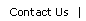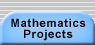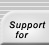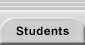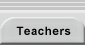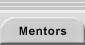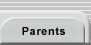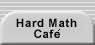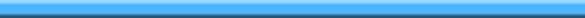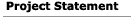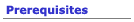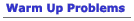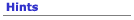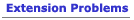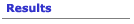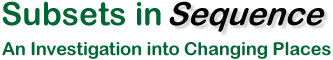The set {a, b, c} has exactly eight subsets. List all the subsets of {a, b, c}. Now arrange those subsets in a sequence so that each subset in the sequence differs from the one before it in one of two ways: One new element is inserted: Example 1: {a}, {a, b} Example 2: {a, b}, {a, b, c} One old element removed Example 1: {a, b, c}, {a, c} Example 2: {b}, Ï Can you create more than one sequence that fits these criteria? Create a listing like this for the subsets of {a, b, c, d}. Describe a general method (or explain why there isn’t one) for creating such a sequence of the subsets of any set {a1, a2, a3, ...an}.Subsets in Sequence Project Description | Prerequisites | Warm Up Problems | Hints | Resources | Teaching Notes | Extensions | Results Making Mathematics Home | Mathematics Projects | Students | Teachers | Mentors | Parents | Hard Math Café |Translations of mathematical formulas for web display were created by tex4ht. © Copyright 2003 Education Development Center, Inc. (EDC)